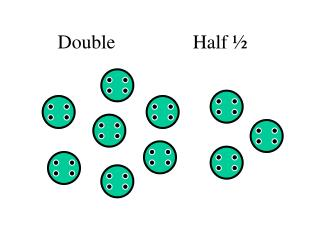DownloadDownload PresentationDouble

# Double

Download Presentation## Double

- - - - - - - - - - - - - - - - - - - - - - - - - - - E N D - - - - - - - - - - - - - - - - - - - - - - - - - - -
##### Presentation Transcript

1. Double Half ½

2. Double Double 8 = 8 + 8 = 8 x 2 = 16

3. Double 12 Double 6 = 6 + 6 = 6 x 2 =

4. Double Double 3 = 3 + 3 = 3 x 2 = 6

5. Find half (½) 5 5 ½ of 10 = 5

6. Find half (½) 5 ½ of 10 =

7. Find Half (½) ½ of 6 = 3